Download Presentation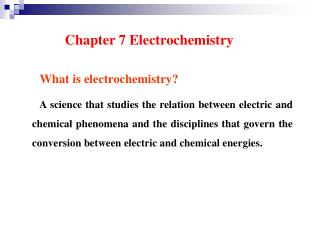Chapter 7 Electrochemistry

# Chapter 7 Electrochemistry - PowerPoint PPT Presentation

Chapter 7 Electrochemistry. What is electrochemistry?. A science that studies the relation between electric and chemical phenomena and the disciplines that govern the conversion between electric and chemical energies. Chapter 7 Electrochemistry. Main contentsI am the owner, or an agent authorized to act on behalf of the owner, of the copyrighted work described.
Download Presentation## Chapter 7 Electrochemistry

An Image/Link below is provided (as is) to download presentation

Download Policy: Content on the Website is provided to you AS IS for your information and personal use and may not be sold / licensed / shared on other websites without getting consent from its author.While downloading, if for some reason you are not able to download a presentation, the publisher may have deleted the file from their server.

- - - - - - - - - - - - - - - - - - - - - - - - - - E N D - - - - - - - - - - - - - - - - - - - - - - - - - -
Presentation Transcript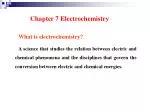Chapter 7 Electrochemistry

What is electrochemistry?

A science that studies the relation between electric and chemical phenomena and the disciplines that govern the conversion between electric and chemical energies.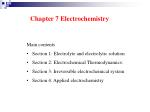Chapter 7 Electrochemistry

• Section 1: Electrolyte and electrolytic solution
• Section 2: Electrochemical Thermodynamics:
• Section 3: Irreversible electrochemical system
• Section 4: Applied electrochemistry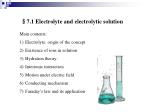§7.1 Electrolyte and electrolytic solution

• Electrolyte: origin of the concept
• Existence of ions in solution
• Hydration theory:
• Interionic interaction
• Motion under electric field
• Conducting mechanism
• Faraday’s law and its application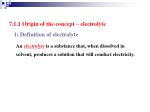7.1.1 Origin of the concept – electrolyte

1) Definition of electrolyte

Anelectrolyte is a substance that, when dissolved in solvent, produces a solution that will conduct electricity.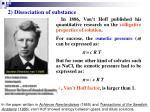2) Dissociation of substance

In 1886, Van’t Hoff published his quantitative research on the colligative properties of solution.

For sucrose, the osmotic pressure () can be expressed as:

 = cR T

But for some other kind of solvates such as NaCl, the osmotic pressure had to be expressed as:

 = i cR T

i, Van’t Hoff factor, is larger than 1.

In the paper written in Achieves Neerlandaises (1885) and Transactions of the Swedish. Academy (1886), van't Hoff showed analogy between gases and dilute solutions.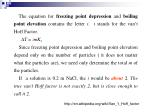The equation for freezing point depression and boiling point elevation contains the letter i. i stands for the van’t Hoff Factor.

∆T = imKf

Since freezing point depression and boiling point elevation depend only on the number of particles ( it does not matter what the particles are), we need only determine the total m of the particles.

If a solution is 0.2 m NaCl, the i would be about 2. The true van’t Hoff factor is not exactly 2, but is close enough to call it 2.

http://en.wikipedia.org/wiki/Van_'t_Hoff_factor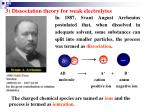

+

+

3) Dissociation theory for weak electrolytes

In 1887, Svant August Arrhenius postulated that, when dissolved in adequate solvent, some substances can split into smaller particles, the process was termed as dissociation.

AB  A+ +B –

molecule cation anion

positive ion negative ion

The charged chemical species are named asions and the process is termed as ionization.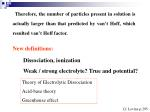Therefore, the number of particles present in solution is actually larger than that predicted by van’t Hoff, which resulted van’t Hoff factor.

New definitions:

Dissociation, ionization

Weak / strong electrolyte? True and potential?

Theory of Electrolytic Dissociation

Acid-base theory

Greenhouse effect

Cf. Levine p.295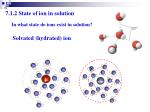Solvated (hydrated) ion

+

7.1.2 State of ion in solution

In what state do ions exist in solution?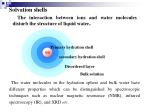Primary hydration shell

secondary hydration shell

Disordered layer

Bulk solution

ion

Solvation shells

The interaction between ions and water molecules disturb the structure of liquid water.

The water molecules in the hydration sphere and bulk water have different properties which can be distinguished by spectroscopic techniques such as nuclear magnetic resonance (NMR), infrared spectroscopy (IR), and XRD etc.Hydration of ion

Coordination number:

Li+: 4, K+: 6

Primary solvation shell:

4-9, 6 is the most common number

Secondary slovation shell:

6-8, for Al3+ and Cr3+: 10-20Na+(g) + Cl(g)

H / kJ mol-1

hydration energy:

784 kJ mol-1

788

784

Na+(aq) + Cl(aq)

4

NaCl(s)

1948, Robinson and Storks

7.1.3 Hydration Theory / Solvation Theory

Why does NaCl only melt at higher temperature, but dissolve in water at room temperature?Long-range forces

The interionic distance for NaCl crystal is 200 pm, while for 0.1 moldm-3 solution is 2000 pm.

To draw Na+ and Cl apart from 200 nm to 2000 nm, the work is: W (/kJ) = 625 / r

for melting: r =1, W = 625 kJ, m.p. = 801 oC。

for dissolution in water: r = 78.5, W = 8 kJ.

Therefore, NaCl is difficult to melt by easy to dissolve in water at room temperature.+

+

+

7.1.4 Interaction between cation and anion

At high concentration

At low concentration

At medium concentration

In equilibrium -- Bjerrum

Cf. Levine, p. 304Owing to the strong interaction, ionic pair forms in concentrated solution.

ionic pair vs free ion

In an ionic pair, the cation and anion are close to each other, and few or no solvent molecules are between them. Therefore, HCl does not ionize and NaCl does not dissociate completely in solvents.Some facts about strong electrolytes

For concentration-dependence of ion pair, see Levine p. 305, Figure 10.10

Degree of association

Activity coefficient is essential for quite dilute solutionsConductor

Charge carrier

samples

1st

electron

Metals, carbonous materials, some metal oxides

2nd

ion

Electrolytic solution, solid-state electrolyte (Al2O3, ZrO2)

3rd

Electron and hole

Semiconductor

4th

polaron

Conducting polymers

5th

electron pair

Superconductors

Mixed conductor

Ion and electron

PbO2, NiOOH

7.1.5 Conducting mechanism of electrolyte

(1) Category of conductor:

Charge carriers:

electron; ion; hole; Cooper electron pair; polaron.+

+

+

+

+

+

+

+

+

I

• Electric transfer of ion in solution under electric field

E

(2) Conducting mechanism

Motion of ions in the solution:

1) diffusion: due to difference in concentration

2) convection: due to the difference in density

3) transfer: due to the effect of electric field

How can current cross the electrode / solution interface ?e

e

H+

Cl

Cl

Cl

H+

e

H+

Cl

e

H+

Cl

H+

H+

H+

e

Cl

Cl

H+

e

Cl

H+

H+

Cl

Cl

At anode:

2Cl  2e  Cl2

At cathode:

2H+ + 2e  H2

Conducting mechanism:

• Transfer of ion in solution under electric field;
• electrochemical reaction at electrode/solution interface.7.1.7 Law of electrolysis

For quantitative electrolysis:

where m is the mass of liberated matter; Q the electric coulomb, z the electrochemical equivalence, F a proportional factor named as Faraday constant, M the molar weight of the matter.

F = (1.6021917  10-19  6.022169  1023 ) C·mol-1

= 96486.69 C·mol-1 usually round off as 96500 C·mol-1, is the charge carried by 1 mole of electron.

Great Britain 1791-1867

Invent the electric motor and generator, and the principles of electrolysis.Current efficiency ()

Current efficiency is lower than 100% due to side-reactions.

For example, evolution of hydrogen occur during electro-deposition of copper.Application of Faraday’s law

1) Definition of ampere:

IUPAC: constant current that would deposit 0.0011180 g of silver per second from AgNO3 solution in one second: 1 ampere.

2) Coulometer: copper / silver / gas (H2, O2) coulometer

3) Electrolytic analysis – electroanalysis

Q ↔m ↔ n ↔ cExercise-1:

A 0.100 molality (mol/kg) solution of NaCl has a freezing-point depression of -0.348 oC, whereas the expected decrease in the freezing point is -0.186 oC. The van’t Hoff factor in this case is 1.87. If there were no ion pairing, we would expect the van’t Hoff factor for NaCl to be 2.00. Similarly, acetic acid in a 0.100 molal solution has a van’t Hoff factor of 1.05. Calculate the concentration of NaCl ion pairs and also the percent ionization of acetic acid form the above information.Exercise-2:

A current of 2.34 A is delivered to an electrolytic cell for 85 min. how many grams of (a) Au from AuCl3, (b) Ag form AgNO3, and (c) Cu from CuCl2 will be plated out?

Exercise-3

Levine: p.317 10. exercise 48

Exercise -4

Yin: p. 217 exercise 1.